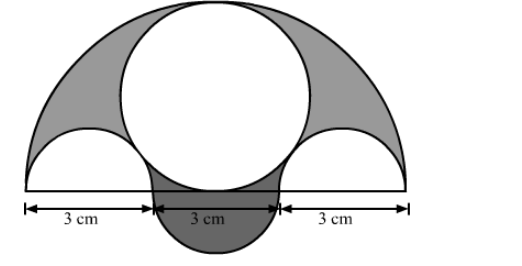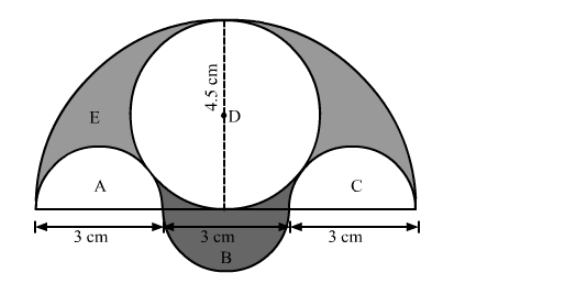# Three semicircles each of diameter 3 cm, a circle of diameter 4.5 cm and a semicircle of radius 4.5 cm`
Question:

Three semicircles each of diameter 3 cm, a circle of diameter 4.5 cm and a semicircle of radius 4.5 cm are drawn in the given figure. Find the area of the shaded region.Solution:= Area of the semi-circle with diameter of 9 cm − Areas of two semi-circles with diameter 3 cm − Area of the circle with diameter 4.5 cm + Area of semi-circle with diameter 3 cm

= Area of the semi-circle with radius of 4.5 cm − 2 × Area of semi-circle with radius 1.5 cm − Area of the circle with radius 2.25 cm + Area of semi-circle with radius 1.5 cm

$=\frac{\pi \times 4.5 \times 4.5}{2}-2 \times \frac{\pi \times 1.5 \times 1.5}{2}-\pi \times 2.25 \times 2.25+\frac{\pi \times 1.5 \times 1.5}{2}$

$=\frac{\pi}{2}(20.25-2.25)-\pi \times 5.0625$

$=\frac{\pi}{2}(18)-\pi \times 5.0625$

$=9 \pi-\pi \times 5.0625$

$=\pi(9-5.0625)$

$=3.9375 \pi$

$=3.9375 \times \frac{22}{7}$

$=12.375 \mathrm{~cm}^{2}$

Thus, the area of the shaded region is $12.375 \mathrm{~cm}^{2}$.